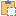#### ProjectPosition Property

Gets or sets the coordinates of a point in the project location coordinate system.

Namespace: Autodesk.Revit.DB
Assembly: RevitAPI (in RevitAPI.dll) Version: 18.0.0.0 (18.0.0.420)

# Syntax

C#
``````[ObsoleteAttribute("This property is deprecated in Revit 2018. Use ProjectLocation.Get/SetProjectPosition() instead.")]
public ProjectPosition this[
XYZ point
] { get; set; }``````
Visual Basic
``````<ObsoleteAttribute("This property is deprecated in Revit 2018. Use ProjectLocation.Get/SetProjectPosition() instead.")> _
Public Property ProjectPosition ( _
point As XYZ _
) As ProjectPosition
Get
Set``````
Visual C++
``````[ObsoleteAttribute(L"This property is deprecated in Revit 2018. Use ProjectLocation.Get/SetProjectPosition() instead.")]
public:
property ProjectPosition^ ProjectPosition[XYZ^ point] {
ProjectPosition^ get (XYZ^ point);
void set (XYZ^ point, ProjectPosition^ value);
}``````

#### Parameters

point
Type: Autodesk.Revit.DB XYZ
The point in model coordinates.

# Remarks

When getting this property, the North/South, East/West, and Elevation values report the coordinates of the point similar to the Revit command "Report Shared Coordinates". To get the values of the transformations applied by this project location, pass XYZ.Zero.

If the project has acquired shared coordinates from a linked model, the shared coordinate transformation will be reflected in the coordinates of the transformed point returned by this property.

When setting this property, the transformations applied to the location are modified such that the passed point becomes the specified North/South, East/West and Elevation, and the coordinate transform will have the designated angular rotation. This is similar to the Revit command "Specify Coordinates at Point".

# ExamplesCopy C#
``````//get and set the value of the projectlocation
public ProjectLocation GetProjectLocation(Autodesk.Revit.DB.Document document)
{
ProjectLocation currentLocation = document.ActiveProjectLocation;

//get the project position
XYZ origin = new XYZ(0, 0, 0);

const double angleRatio = Math.PI / 180;   // angle conversion factor

ProjectPosition projectPosition = currentLocation.GetProjectPosition(origin);
//Angle from True North
double angle = 30.0 * angleRatio;   // convert degrees to radian
double eastWest = 30.0;     //East to West offset
double northSouth = 23.32;   //North to South offset
double elevation = 22.14;    //Elevation above ground level

//create a new project position
ProjectPosition newPosition =
document.Application.Create.NewProjectPosition(eastWest, northSouth, elevation, angle);

if (null != newPosition)
{
//set the value of the project position
currentLocation.SetProjectPosition(origin, newPosition);
}

return currentLocation;
}``````Copy VB.NET
``````'get and set the value of the projectlocation
Public Function GetProjectLocation(document As Autodesk.Revit.DB.Document) As ProjectLocation
Dim currentLocation As ProjectLocation = document.ActiveProjectLocation

'get the project position
Dim origin As New XYZ(0, 0, 0)

Const angleRatio As Double = Math.PI / 180
' angle conversion factor
Dim projectPosition As ProjectPosition = currentLocation.GetProjectPosition(origin)
'Angle from True North
Dim angle As Double = 30.0 * angleRatio
Dim eastWest As Double = 30.0
'East to West offset
Dim northSouth As Double = 23.32
'North to South offset
Dim elevation As Double = 22.14
'Elevation above ground level
'create a new project position
Dim newPosition As ProjectPosition = document.Application.Create.NewProjectPosition(eastWest, northSouth, elevation, angle)

If newPosition IsNot Nothing Then
'set the value of the project position
currentLocation.SetProjectPosition(origin, newPosition)
End If

Return currentLocation
End Function``````